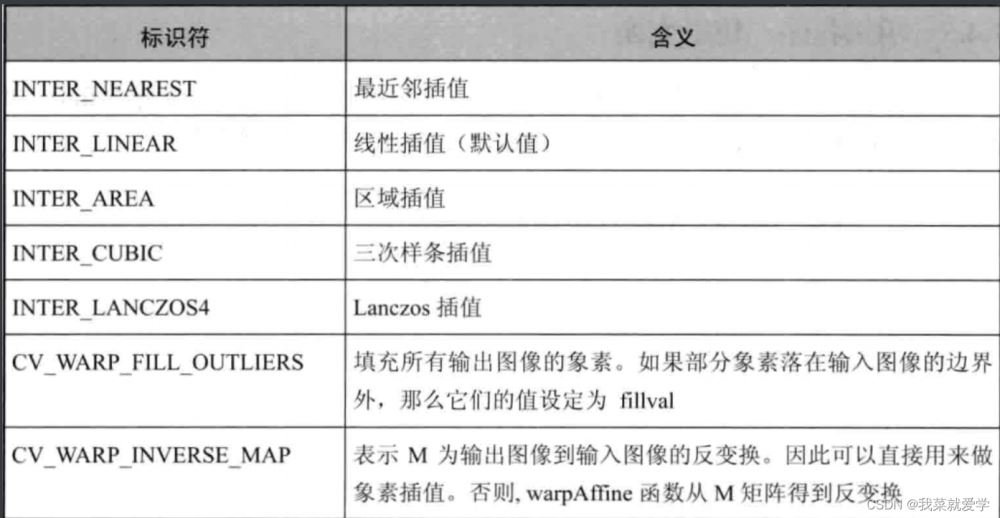﻿ OpenCV仿射变换的示例代码_python_脚本之家
python# OpenCV仿射变换的示例代码

## 1、认识仿射变换

• 旋转：ratation(线性变换)
• 平移：translation(向量加)
• 缩放：scale(线性变换)## 2、仿射变换的求法

• 已知X和T，而且已知它们是有联系的，接下来的跟着就是求出矩阵M。
• 已知M和X，想要求得T。只要应用算式T=M*X即可。## 3、进行仿射变换：warpAffine()函数

warpAffine()函数的作用依据下面的公式对图像做仿射变换：`void warpAffine(InputArray src,OutputArray dst,InputArray M,Size dsize,int flags=INTER_LINEAR,intborderMOde=BODER_CONSTANT,const Scalar& borderValue=Scalar())`
• 第一个参数：输入图像
• 第二个参数：输出图像，函数调用后的运算结果存在这里，需要和源图片有一样的尺寸和类型
• 第三个参数：2 x 3的变换矩阵，求得的仿射变换
• 第四个参数：表示输出图像的尺寸
• 第五个参数：插值方法的标识符。默认值是线性插值法（INTER_LINEAR）• 第六个参数：边界像素模式
• 第七个参数：在恒定的边界情况下取值，默认值Scalar()，即0

## 4、计算二维旋转变换矩阵：getRotationMatrix2D()函数

`Mat getRotationMatrix2D(Point2f center,double angle,double scale)`
• 第一个参数：表示源图像的旋转中心
• 第二个参数：旋转角度。角度为正值表示向逆时针旋转（坐标原点是左上角）
• 第三个参数：缩放系统

## 5、示例程序：

```#include<opencv2/opencv.hpp>
#include<opencv2/imgproc/imgproc.hpp>
#include<opencv2/highgui/highgui.hpp>
#include<iostream>
using namespace std;
using namespace cv;
#define WINDOW_NAME1 "【原始图窗口】"
#define WINDOW_NAME2 "【经过Warp后的窗口】"
#define WINDOW_NAME3 "【经过Warp和Rotate后的窗口】"
int main()
{
system("color 2F");
//参数准备
Point2f srcTriangle;
Point2f dstTriangle;

Mat rotMat(2, 3, CV_32FC1);
Mat warpMat(2, 3, CV_32FC1);
Mat srcImage, dstImage_warp, dstImage_warp_rotate;
//加载源图像
//设置目标图像的大小和类型与源图像一致
dstImage_warp = Mat::zeros(srcImage.rows, srcImage.cols, srcImage.type());
//设置源图像和目标图像上的三组点以计算仿射变换
//srcTriangle = Point2f(0, 0); //Point2f表示Point类的两个数据x,y为float类型；vector 表示存放四维int
//srcTriangle = Point2f(static_cast<float>(srcImage.cols - 1), 0);
//srcTriangle = Point2f(0, static_cast<float>(srcImage.rows - 1));
//dstTriangle = Point2f(static_cast<float>(srcImage.cols*0.0), static_cast<float>(srcImage.rows*0.33));
//dstTriangle = Point2f(static_cast<float>(srcImage.cols*0.65), static_cast<float>(srcImage.rows*0.35));
//dstTriangle = Point2f(static_cast<float>(srcImage.cols*0.15), static_cast<float>(srcImage.rows*0.6));
//获取变换矩阵，指定三个点
srcTriangle = Point2f(50, 50);
srcTriangle = Point2f(200, 50);
srcTriangle = Point2f(50, 200);
dstTriangle = Point2f(100, 100);
dstTriangle = Point2f(200, 50);
dstTriangle = Point2f(100, 250);
//求仿射变换,得到一个2x3的矩阵
warpMat = getAffineTransform(srcTriangle, dstTriangle);
//对源图像应用刚刚的求得的仿射变换
warpAffine(srcImage, dstImage_warp, warpMat, dstImage_warp.size());

//对图像进行缩放后再旋转
//计算图像中点顺时针旋转50°缩放因子为0.6的旋转矩阵
Point center = Point(dstImage_warp.cols / 2, dstImage_warp.rows / 2);
double angle = -30.0;
double scale = 0.8;
//通过上面的旋转细节信息求出旋转矩阵
rotMat = getRotationMatrix2D(center, angle, scale);
//旋转已经缩放后的图像
warpAffine(dstImage_warp, dstImage_warp_rotate, rotMat, dstImage_warp.size());
imshow(WINDOW_NAME1, srcImage);
imshow(WINDOW_NAME2, dstImage_warp);
imshow(WINDOW_NAME3, dstImage_warp_rotate);
waitKey(0);
return 0;

}
```